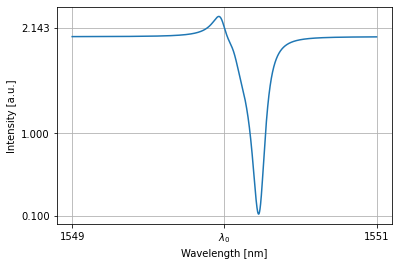# Optimize an optical readout based on ring resonators¶

## Imports¶

:

import torch
import numpy as np
import matplotlib.pyplot as plt
from tqdm.notebook import tqdm, trange
from numpy.fft import fft, ifft, fftfreq
from scipy.signal import butter, lfilter

import photontorch as pt

torch.manual_seed(33)
np.random.seed(34)


## Parameters¶

:

cuda = torch.cuda.is_available()
bitrate = 50e9 # bps
dt = 1e-14 # new sampling timestep
samplerate = 1/dt # new sampling rate
angles = np.pi*np.array([0.5,0,-0.5,-0.5,-0.5,1,1]) # output angles of the output waveguides
power = 1e-3 #[W]
latencies = np.arange(0.01,2.5,0.1)
num_bits = 500

c = 299792458.0 #[m/s] speed of light
neff = 2.86 # effective index
ng = 3.0 # group index of waveguide
wl0 = 1.55e-6

# Set global environment
environment = pt.Environment(
wl=np.linspace(1.549e-6,1.551e-6,10000),
freqdomain=True,
)
pt.set_environment(environment);
pt.current_environment()

:

key value description
nameenvname of the environment
t0.000e+00[s] full 1D time array.
t00.000e+00[s] starting time of the simulation.
t1None[s] ending time of the simulation.
num_t1number of timesteps in the simulation.
dtNone[s] timestep of the simulation
samplerateNone[1/s] samplerate of the simulation.
bitrateNone[1/s] bitrate of the signal.
bitlengthNone[s] bitlength of the signal.
wl[1.549e-06, 1.549e-06, ..., 1.551e-06][m] full 1D wavelength array.
wl01.549e-06[m] start of wavelength range.
wl11.551e-06[m] end of wavelength range.
num_wl10000number of independent wavelengths in the simulation
dwl2.000e-13[m] wavelength step sizebetween wl0 and wl1.
f[1.935e+14, 1.935e+14, ..., 1.933e+14][1/s] full 1D frequency array.
f01.935e+14[1/s] start of frequency range.
f11.933e+14[1/s] end of frequency range.
num_f10000number of independent frequencies in the simulation
df-2.499e+07[1/s] frequency step between f0 and f1.
c2.998e+08[m/s] speed of light used during simulations.
freqdomainTrueonly do frequency domain calculations.

## Single Weight (AllPass filter)¶

First we define a simple all pass filter as a network:

:

class AllPass(pt.Network):
def __init__(self, extra_ring_phase=0):
super(AllPass, self).__init__()
ring_length = 425.8734943010671*wl0/ng # on resonance
self.dc = pt.DirectionalCoupler(coupling=0.1, trainable=False)
self.wg = pt.Waveguide(length=ring_length, loss=1500, neff=neff, ng=ng, wl0=wl0, phase=extra_ring_phase, trainable=True)
self.wg_out = pt.Waveguide(length=0, loss=0, neff=neff, ng=ng, wl0=wl0, phase=2*np.pi*np.random.rand(), trainable=False)


We see that this allpass filter is on resonance:

:

with pt.Network() as nw:
nw.ap = AllPass()
nw.s = pt.Source()
nw.d = pt.Detector()

detected = nw(source=1)
nw.plot(detected)

plt.xticks([1549,wl0*1e9,1551], [1549,'$\lambda_0$',1551]); plt.yticks([0.1,1])
plt.grid(True)
plt.show()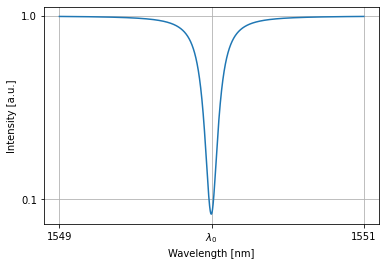## Train Network¶

We can train this all-pass filter to have a specific transmission.

:

target = torch.tensor(0.9)
optimizer = torch.optim.Adam(nw.parameters(), lr=0.03)
lossfunc = torch.nn.MSELoss()

rng = trange(400)
with pt.Environment(wl=wl0, freqdomain=True, grad=True):
for i in rng:
result = nw(source=1)[-1,0,0,0] # last timestep, only wavelength, only detector, only batch
loss = lossfunc(result, target)
loss.backward()
optimizer.step()
rng.set_postfix(result='%.6f'%result.item())

detected = nw(source=1)
nw.plot(detected)
plt.xticks([1549,wl0*1e9,1551], [1549,'$\lambda_0$',1551]); plt.yticks([0.1,1])
plt.grid(True)
plt.show()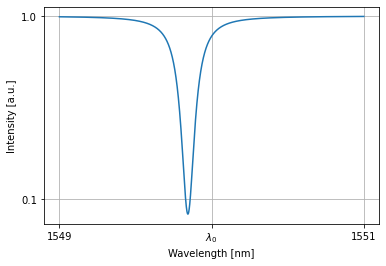## Multiple Weights (Collection of AllPass Filters)¶

:

class MultipleAllPasses(pt.Network):
def __init__(self, num_allpasses):
super(MultipleAllPasses, self).__init__()
for i in range(num_allpasses):


All rings in the allpass collections are initialized close to resonance:

:

nw = MultipleAllPasses(4)
nw.plot(nw(source=1))
plt.xticks([1549,wl0*1e9,1551], [1549,'$\lambda_0$',1551]); plt.yticks([0.1,1])
plt.grid(True)
plt.show()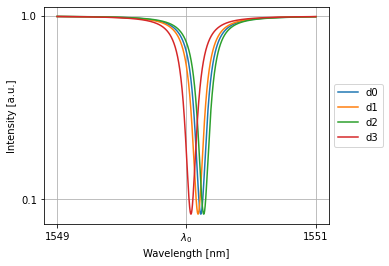## Train Network¶

We can train this collection of allpasses to have any kind of weight:

:

target = torch.tensor([0.9,0.5,0.1,0.7])
optimizer = torch.optim.Adam(nw.parameters(), lr=0.03)
lossfunc = torch.nn.MSELoss()

rng = trange(400)
with pt.Environment(wl=wl0, freqdomain=True, grad=True):
for i in rng:
result = nw(source=1)[-1,0,:,0] # last timestep, only wavelength, all detectors, only batch
loss = lossfunc(result, target)
loss.backward()
optimizer.step()
rng.set_postfix(loss='%.10f'%loss.item())

print('weights:')
print(' '.join(['%.2f'%w for w in nw(source=1)[-1,0,:,0]]))

nw.plot(nw(source=1))
plt.xticks([1549,wl0*1e9,1551], [1549,'$\lambda_0$',1551]); plt.yticks([0.1,1])
plt.grid(True)
plt.show()


weights:
0.90 0.50 0.10 0.70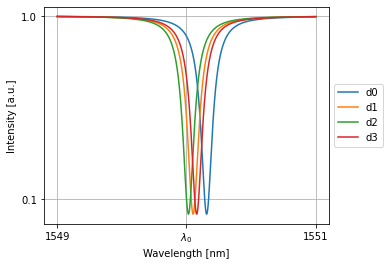## Combination of Multiple Weights (Readout)¶

:

class Combiner(pt.Component):
def __init__(self, num_inputs, name=None):
self.num_inputs = num_inputs
self.num_ports = num_inputs + 1
pt.Component.__init__(self, name=name)
def set_S(self, S):
S[0, :, :self.num_inputs, -1] = 1
S[0, :, -1, :self.num_inputs] = 1

:

class Readout(pt.Network):
def __init__(self, num_weights):
self.allpasses = MultipleAllPasses(num_allpasses=num_weights)
self.det = pt.Detector()
self.combiner = Combiner(num_weights)
for i in range(num_weights):


This ring combines multiple inputs with different weights:

:

nw = Readout(4)
with pt.Environment(wl=wl0, freqdomain=True):
print('weights:')
print(' '.join(['%.2f'%w for w in nw.allpasses(source=1)[-1,0,:,0]]))

nw.plot(nw(source=1))
plt.xticks([1549,wl0*1e9,1551], [1549,'$\lambda_0$',1551]); plt.yticks([0.1,1])
plt.grid(True)
plt.show()

weights:
0.56 0.76 0.73 0.84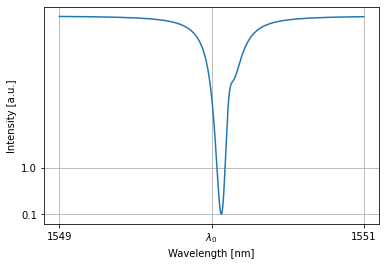:

src = torch.randn(4).rename("s") + 0.3 # lower dimensional sources need to have named dimensions.
target = torch.tensor(2.14325, dtype=torch.float32)
optimizer = torch.optim.Adam(nw.allpasses.parameters(), lr=0.03)
lossfunc = torch.nn.MSELoss()

rng = trange(400)
with pt.Environment(wl=wl0, freqdomain=True, grad=True):
for i in rng:
result = nw(source=src)[-1,0,0,0] # last timestep, only wavelength, only detector, only batch
loss = lossfunc(result, target)
loss.backward()
optimizer.step()
rng.set_postfix(loss='%.10f'%loss.item())

weights = nw.allpasses(source=1)[-1,0,:,0]
print('weights:')
print('+'.join(['%.2f'%w for w in weights])+'%.2f'%weights.sum().item())

nw.plot(nw(source=src))
plt.xticks([1549,wl0*1e9,1551], [1549,'$\lambda_0$',1551]); plt.yticks([0.1,1, target.item()])
plt.grid(True)
plt.show()


weights:
0.95+0.10+0.66+0.712.42